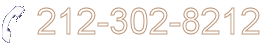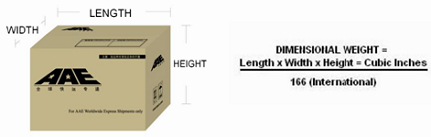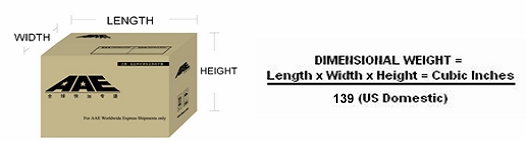Learn(Shipping 101)
FAQs

FAQ :How to calculate dimensional weight? : According to IATA regulations, air express dimensional weight is calculated as illustrated below. For International Air Express Shipments - Calculate the volume of the parcel by multiplying the carton's dimensions (length x width x height), then divide it by the dimensional weight factor of 166 if measured in inches. If measured in centimeters, the dimensional weight factor would be 6000, and the result of dimensional weight would be in kilos. Multiply kilos by 2.20462 to convert to pounds. Example: a carton with the dimensions of 40"(L) x 30"(W) x 20"(H) = 24,000 cubic inches, divided by 166 = 144.58 pounds for a "dimensional weight" of 145 pounds (always round to the next full pound).For International Air Express Shipments - Calculate the volume of the parcel by multiplying the carton's dimensions (length x width x height), then divide it by the dimensional weight factor of 139 if measured in inches. If measured in centimeters, the dimensional weight factor would be 7009, and the result of dimensional weight would be in kilos. Multiply kilos by 2.20462 to convert to pounds. Example: a carton with the dimensions of 40"(L) x 30"(W) x 20"(H) = 24,000 cubic inches, divided by 139 = 172.66 pounds for a "dimensional weight" of 173 pounds (always round to the next full pound).